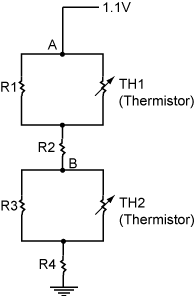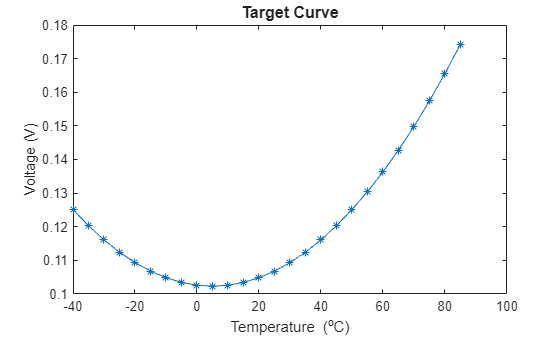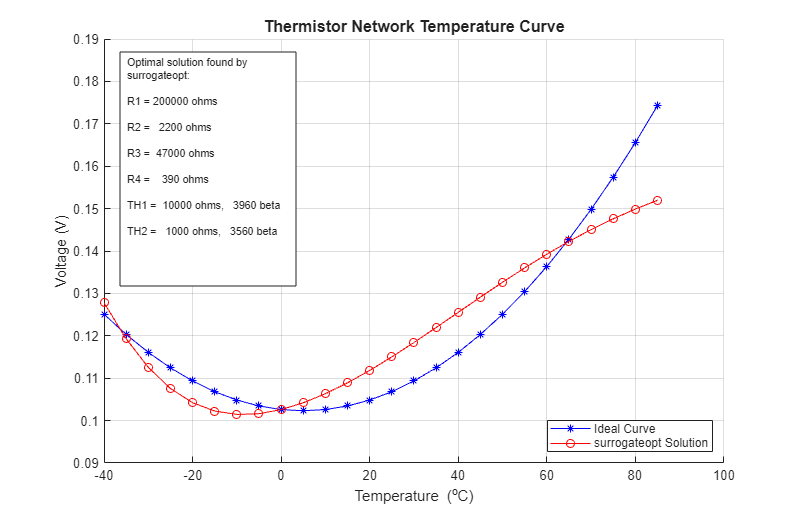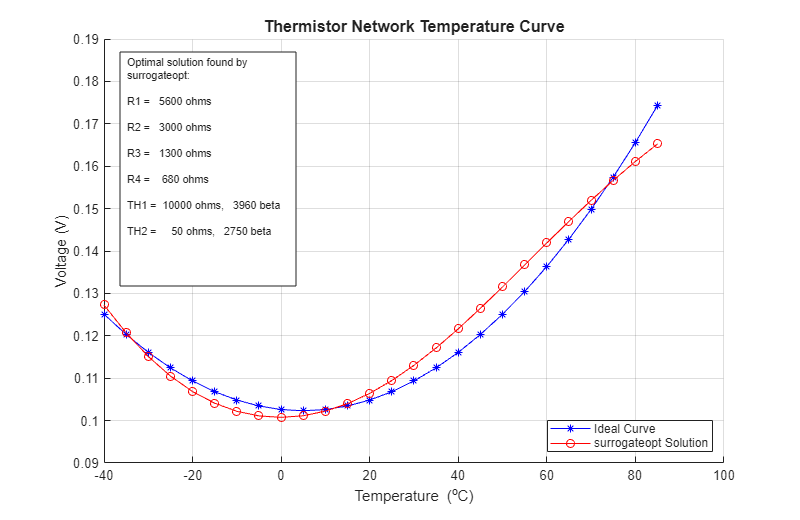# Integer Optimization with Custom Output Function

This example shows how to choose the resistors and thermistors in a circuit to best match a specified curve at one point in the circuit. You must choose all of the electronic components from a list of available components, which means this is a discrete optimization problem. To help visualize the progress of the optimization, the example includes a custom output function that displays the quality of the intermediate solutions as the optimization progresses. Because this is an integer problem with a nonlinear objective function, use the `surrogateopt` solver.

This example is adapted from Lyon .

### Problem Description

The problem involves this circuit.A voltage source holds point A at 1.1V. The problem is to select resistors and thermistors from a list of standard components so that the voltage at point B matches the target curve as a function of temperature.

```Tdata = -40:5:85; Vdata = 1.026E-1 + -1.125E-4 * Tdata + 1.125E-5 * Tdata.^2; plot(Tdata,Vdata,'-*'); title('Target Curve','FontSize',12); xlabel('Temperature (^oC)'); ylabel('Voltage (V)')````load StandardComponentValues`

The `Res` vector contains the standard resistor values. The `ThBeta` and `ThVal` vectors contain standard parameters for the thermistors. Thermistor resistance as a function of temperature $T$ is

`${R}_{Th}=\frac{{R}_{25}}{\mathrm{exp}\left(\beta \frac{T-{T}_{25}}{T\cdot {T}_{25}}\right)}.$`

• ${R}_{Th}$ is the thermistor resistance.

• ${R}_{25}$ is the resistance at 25 degrees Celsius, parameter `ThVal`.

• ${T}_{25}$ is the temperature 25 degrees Celsius.

• $T$ is the current temperature.

• $\beta$ is the thermistor parameter `ThBeta`.

Based on standard voltage calculations, the equivalent series values of the resistances of the ${R}_{1}-T{h}_{1}$ block is

${R}_{1}^{equivalent}=\frac{{R}_{1}{Th}_{1}}{{R}_{1}+{Th}_{1}}$,

and the equivalent resistance of the ${R}_{3}-T{h}_{2}$ block is

${R}_{3}^{equivalent}=\frac{{R}_{3}{Th}_{2}}{{R}_{3}+{Th}_{2}}$.

Therefore, the voltage at point B is

$V=1.1\frac{{R}_{3}^{equivalent}+{R}_{4}}{{R}_{1}^{equivalent}+{R}_{2}+{R}_{3}^{equivalent}+{R}_{4}}$.

### Convert Problem to Code

The problem is to choose resistors ${R}_{1}$ through ${R}_{4}$ and thermistors $T{h}_{1}$ and $T{h}_{2}$ so that the voltage $V$ best matches the target curve. Have the control variable x represent these values:

• `x(i)` = index of ${R}_{i}$, for `i` from 1 through 4

• `x(5)` = index of $T{h}_{1}$

• `x(6)` = index of $T{h}_{2}$

The `tempCompCurve` function calculates the resulting voltage in terms of `x` and the temperature `Tdata`.

`type tempCompCurve`
```function F = tempCompCurve(x,Tdata) %% Calculate Temperature Curve given Resistor and Thermistor Values % Copyright (c) 2012-2019, MathWorks, Inc. %% Input voltage Vin = 1.1; %% Thermistor Calculations % Values in x: R1 R2 R3 R4 RTH1(T_25degc) Beta1 RTH2(T_25degc) Beta2 % Thermistors are represented by: % Room temperature is 25degc: T_25 % Standard value is at 25degc: RTHx_25 % RTHx is the thermistor resistance at various temperatures % RTH(T) = RTH(T_25degc) / exp (Beta * (T-T_25)/(T*T_25)) T_25 = 298.15; T_off = 273.15; Beta1 = x(6); Beta2 = x(8); RTH1 = x(5) ./ exp(Beta1 * ((Tdata+T_off)-T_25)./((Tdata+T_off)*T_25)); RTH2 = x(7) ./ exp(Beta2 * ((Tdata+T_off)-T_25)./((Tdata+T_off)*T_25)); %% Define equivalent circuits for parallel Rs and RTHs R1_eq = x(1)*RTH1./(x(1)+RTH1); R3_eq = x(3)*RTH2./(x(3)+RTH2); %% Calculate voltages at Point B F = Vin * (R3_eq + x(4))./(R1_eq + x(2) + R3_eq + x(4)); ```

The objective function is the sum of squares of the differences between the target curve and the resulting voltages for a set of resistors and thermistors, over the target range of temperatures.

`type objectiveFunction`
```function G = objectiveFunction(x,StdRes, StdTherm_Val, StdTherm_Beta,Tdata,Vdata) %% Objective function for the thermistor problem % Copyright (c) 2012-2019, MathWorks, Inc. % % StdRes = vector of resistor values % StdTherm_val = vector of nominal thermistor resistances % StdTherm_Beta = vector of thermistor temperature coefficients % Extract component values from tables using integers in x as indices y = zeros(8,1); x = round(x); % in case of noninteger components y(1) = StdRes(x(1)); y(2) = StdRes(x(2)); y(3) = StdRes(x(3)); y(4) = StdRes(x(4)); y(5) = StdTherm_Val(x(5)); y(6) = StdTherm_Beta(x(5)); y(7) = StdTherm_Val(x(6)); y(8) = StdTherm_Beta(x(6)); % Calculate temperature curve for a particular set of components F = tempCompCurve(y, Tdata); % Compare simulated results to target curve Residual = F(:) - Vdata(:); Residual = Residual(1:2:26); %% G = Residual'*Residual; % sum of squares ```

### Monitor Progress

To observe the progress of an optimization, call an output function that plots the best response of the system found so far and the target curve. The `SurrOptimPlot` function plots these curves, and updates the curves only when the current objective function value decreases. This custom output function is lengthy, so it is not shown here. To see the content of this output function, enter `type SurrOptimPlot`.

### Optimize Problem

To optimize the objective function, use `surrogateopt`, which accepts integer variables. First, set all variables to be integer.

`intCon = 1:6;`

Set the lower bounds on all variables to 1.

`lb = ones(1,6);`

The upper bounds for the resistors are all the same. Set the upper bounds to the number of entries in the `Res` data.

`ub = length(Res)*ones(1,6);`

Set the upper bounds for the thermistors to the number of entries in the `ThBeta` data.

`ub(5:6) = length(ThBeta)*[1,1];`

Set options to use the `SurrOptimPlot` custom output function, and to use no plot function. Also, to protect against possible interruptions of the optimization, specify a checkpoint file named `'checkfile.mat'`.

```options = optimoptions('surrogateopt','CheckpointFile','C:\TEMP\checkfile.mat','PlotFcn',[],... 'OutputFcn',@(a1,a2,a3)SurrOptimPlot(a1,a2,a3,Tdata,Vdata,Res,ThVal,ThBeta));```

To give the algorithm a better initial set of points to search, specify a larger initial random sample than the default.

`options.MinSurrogatePoints = 50;`

Run the optimization.

```rng default % For reproducibility objconstr = @(x)objectiveFunction(x,Res,ThVal,ThBeta,Tdata,Vdata); [xOpt,Fval] = surrogateopt(objconstr,lb,ub,intCon,options);``````Surrogateopt stopped because it exceeded the function evaluation limit set by 'options.MaxFunctionEvaluations'. ```

### Optimize with More Function Evaluations

To attempt to get a better fit, restart the optimization from the checkpoint file, and specify more function evaluations. This time, use the `surrogateoptplot` plot function to monitor the optimization process more closely.

```clf % Clear previous figure opts = optimoptions(options,'MaxFunctionEvaluations',600,'PlotFcn','surrogateoptplot'); [xOpt,Fval] = surrogateopt('C:\TEMP\checkfile.mat',opts);``````Surrogateopt stopped because it exceeded the function evaluation limit set by 'options.MaxFunctionEvaluations'. ```

Using more function evaluations improves the fit slightly.

### References

 Lyon, Craig K. Genetic algorithm solves thermistor-network component values. EDN Network, March 19, 2008. Available at `https://www.edn.com/design/analog/4326942/Genetic-algorithm-solves-thermistor-network-component-values`.